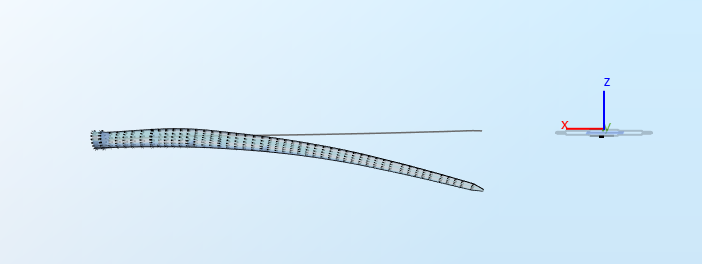## 1 Test description

In this test we compare the eigenfrequencies of an isolated IEA 15-MW blade given in Table 2.1 of Gaertner et al. (2020) to results obtained in Ashes.

## 2 Model

The IEA 15-MW blade can be found in the Blade database. The blade is fixed at its root.

## 3 Benchmarks

In the following sections, the different modeshapes are illustrated and the expected eigenfrequencies as listed in Gaertner et al. (2020) are given.

### 3.1 First flapwise mode

The expected eigenfrequency is
$$f_1 = 0.555 \text{ Hz}$$
. The mode shape is illustrated in the figure below:### 3.2 First edgewise mode

The expected eigenfrequency is
$$f_2 =0.642\text{ Hz}$$
. The mode shape is illustrated in the figure below:## 4 Results

The test is considered passed if the results from Ashes are within 5% of the data from the specification document. A bar plot comparing the eigenfrequncies is available on the following link: